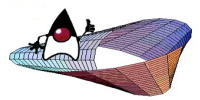numEclipse - An Eclipse based workbench for

Numerical Computing

# Calculus

This short chapter introduces some basic functions related to numerical differentiation and integration.

## 8.1 Differentiation

numEclipse implements three numerical differentiation functions, i.e., diff, gradient and del2.

### diff

diff is used to calculate the forward differences of a function values given in a vector or a matrix.

d = diff(Y)

It calculates the first order forward differences. If Y is a vector of length l, then it returns a vector of length n - 1 such that d(i) = y(i+1) - y(i). If X is a matrix of size (k, l) then it returns a matrix of size (k-1, l) such that d(i, j) = y(i + 1, j) = y(i, j).

dn = diff(Y, n)

The second option calculates the nth order forward differences. If Y is a vector of length l, then it returns a vector of length l - n such that dn(i) = dn-1(i+1) - dn-1(i) and d0(i) = y(i).

If X is a matrix of size (k, l) then it returns a matrix of size (k-n, l) such that dn(i, j) = dn-1(i+1, j) - dn-1(i, j) and d0(i, j) = y(i, j).

dn = diff(Y, n, dim)

This variation of the function works like the previous alternatives except that the difference are calculated along the dimension specified by the third argument dim. The legal values of dim are 1 (row-wise) and 2 (column-wise).

> x = rand(3, 4)

x =

0.5391 0.6476 0.9679 0.5680
0.2393 0.0580 0.8823 0.7728
0.0951 0.0122 0.2702 0.4076

> diff (x,1, 1)

ans =

-0.2997 -0.5897 -0.0857 0.2048
-0.1442 -0.0458 -0.6121 -0.3652

> diff (x, 2, 2)

ans =

0.2117 -0.7202
1.0056 -0.9338
0.3409 -0.1206

In the above example, we create a random matrix of size (3, 4). Then we show the first order differences along the rows, diff(x, 1, 1). Then, we show the second order differences along columns, diff(x, 2, 2).

gradient of a function calculates the direction of increasing value of the function.

[fx fy] = gradient(f)

If f is a vector, then gradient calculates the one dimensional numerical gradient of f. In case of a matrix, it returns the x and y components of the gradient, i.e., fx, fy.

> f = rand(3, 4)

f =

0.2428 0.9297 0.3220 0.5276
0.2732 0.2451 0.1186 0.3434
0.5275 0.6650 0.1976 0.8577

> [fx, fy] = gradient(f)

fx =

0.6869 0.0396 -0.2010 0.2056
-0.0281 -0.0773 0.0492 0.2248
0.1375 -0.1650 0.0964 0.6601

fy =

0.0304 -0.6846 -0.2034 -0.1842
0.1424 -0.1323 -0.0622 0.1651
0.2543 0.4199 0.0790 0.5143

numEclipse adopted the implementation from GNU Octave.

### del2

del2 calculates the finite difference approximation of Laplace's differential operator.

L = del2(U)

L is a matrix of same size as U, such that each element in L is a difference of corresponding element in X with the average of its four neighbouring elements.

L = del2(X, h)

Here h is the spacing spacing at each point.

L = del2(X, hx, hy)

Here hx and hy are spacings in x and y direction at each point.

> u = rand(4)

u =

0.5124 0.6993 0.8292 0.2036
0.8024 0.2478 0.6449 0.0626
0.2812 0.2102 0.4668 0.8694
0.9626 0.7843 0.3583 0.0825

> l = del2(u, 0.1, 0.2)

l =

-6.4945 1.1620 -18.8502 -12.9650
18.7224 26.3790 -24.4466 -18.5615
15.7072 12.0146 4.0869 -6.3098
8.8410 1.4557 4.6235 -16.1699

numEclipse adopted the implementation from GNU Octave.

## 8.2 Integration

numEclipse implements three numerical integration functions, i.e., quad, quadl and dblquad.

This method evaluates the numerical integral of a given function using adaptive Simpson method.

I = quad(func, a, b)

I = quad(func, a, b, tol)

The first function call approximates the definite integral of function func from a to b with error tolerance 1e-6. The second alternative allows a user-defined tolerance in the last argument.

> f = @sin

f =

@sin

> quad(f, 0, pi)

ans =

2.0000

numEclipse adopted the implementation from the paper "Adaptive Quadrature - Revisited" by  Gander, W. This paper is available on the internet at http:// www.inf.ethz.ch/personal/gander.

This function is a variation of the above. It approximates the numerical integral using adaptive Lobatto quadrature.

I = quadl(func, a, b)

I = quadl(func, a, b, tol)

The first function call approximates the definite integral of function func from a to b with error tolerance 1e-6. The second alternative allows a user-defined tolerance in the last argument.

> f = @sqrt

f =

@sqrt

> quadl(f, 0, pi)

ans =

3.7122

numEclipse adopted the implementation from the paper "Adaptive Quadrature - Revisited" by  Gander, W. This paper is available on the internet at http:// www.inf.ethz.ch/personal/gander.

dblquad approximates the double numerical integral of a given function.

I = dblquad(func, xmin, xmax, ymin, ymax)

I = dblquad(func, xmin, xmax, ymin, ymax, tol)

The first function call approximates the definite double integral of function func(x, y) for x from xmin to xmax and y from ymin and ymax with error tolerance 1e-6. The second alternative allows a user-defined tolerance in the last argument.

function z = func2(x, y)
z = x.*x + y.*y;

> I = dblquad(@func2, 0, 1, 0, 1, 1e-8)

I =

0.6667

The implementation of this function is based on Gaussian Quadrature on rectangular elements and code is adopted from the book "Numerical Methods in Engineering with MATLAB" by Jaan Kiusalaas.